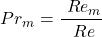# Prandtl Number – Calculation and Effective Use

#### Engineering

The Prandtl Number is an unitless ratio that describes the momentum diffusivity to thermal diffusivity of a liquid. Using a Prandtl number provides the relation of the thickness of the velocity and the liquids thermal boundary. Materials that have a smaller Prandtl number tend to have higher thermal conductivity and are a stronger choice when choosing a heat conducting material. In this article, you will learn the origin of the number, how to calculate the Prandtl number, values of everyday materials, and how to use it effectively.

## Origin of the Prandtl number

The number is named after Ludwig Prandtl, a German scientist made famous for his ideas surrounding fluids and their boundary layers. His work provided a great breakthrough in the way mechanical and thermal fluid properties were analyzed. His contributions also inspired the Schmidt number and Lewis number which are other dimensionless ratios describing fluid properties.

## How to Calculate a Prandtl Number

The Prandtl number is comprised of a laminar calculation and a turbulent calculation.

### Laminar Calculations

There are two ways to go about calculating a Prandtl Number(The Laminar part). The first and most simple way is if you already have the momentum diffusivity(υ) and heat diffusivity(α) of the material in question. Then you simply divide the momentum diffusivity by the heat diffusivity to get the value. ​The second way to calculate it is by using the specific heat(cp), the dynamic viscosity(μ), and thermal conductivity(k) of the material. Next, multiply the specific heat and dynamic viscosity and then divide by the thermal conductivity.### Turbulent Calculations

There is also a turbulent part to the equation. Calcuate this part by obtaining the turbulent momentum diffusivity(υt) and dividing it by the turbulent heat diffusivity(αt).### Effective Calculations

Once the laminar part and the turbulent part are obtained, simply add them together. This produces a measurement called an effective Prandtl number.### Magnetic Calculations

Lastly there is the magnetic variation that comes from either dividing the momentum diffusivity(υ) by the magnetic diffusivity(η), or can also come from dividing the magnetic Reynolds number(Rem) by the normal Reynolds number(Re).## The Parts of a Effective Prandtl Number

The most accurate way to calculate a Prandtl number is by considering both the laminar and turbulent parts of the number. This is comprised of two main parts the laminar part and the turbulent part. The laminar partthe number describes the fluid’s even and steady flow. The turbulent part describes the irregular part of the flow, when the fluid is encountering stress, and changes in direction and speed. It is important to account for both of these in the calculations. One of the best ways to see the turbulent application is in Reynolds analogy which is good at showing the relationship between heat transfer and turbulent momentum. It uses an experimental material with an approximate 1.0 turbulent Prandtl number. This shows a similar momentum diffusivity and heat diffusivity.

## Uses of a Prandtl Number

One of the most practical uses of a Prandtl number is being able to tell what the specific material does best. The number describes the specific fluid properties of the material. Specifically, when the number is less than one it shows that the material diffuses heat much faster than it can move. This means that a material with a smaller number is a better choice for a heat-conducting liquid. However, with a bigger value, the substance itself diffuses at a much faster rate than the heat transfer rate meaning that heat moves slower throughout the material. Moreover, the specification of everyday materials derives directly from the Prandtl Number. Oil used in generators and engines is one such example. Because the oil has a very large value it’s able to prioritize the expansion of the material over then heat transfer properties.

Another use of the number is it’s use in calculations. For instance, it is used in finding a Lewis number, free and forced convection, and heat transfer calculations. The other variations of the number also have good uses. For instance, the magnetic Prandtl number shows the magnetism to gaseous/liquid states in planets and stars. This has helped us discover more about the inner workings of stars and celestial gaseous bodies. Specifically, it provides insight into the magnetic and electrical influences working fluids have on massive gaseous bodies.